## How to program in Python + Paste Code for review/help

Post

### Re: How to program in Python + Paste Code for review/help

#46
Just some nice code for generating IP Addresses.
No error catching, so for some reason, typing "sd" instead of a number gives some crazy errors (details below) unless I add "sd = ' ' " at the start.
As Grumblesaur pointed out, input returns a string, so why if I enter "sd", does it crash out?

This is the working code - copy/paste - run in python, it works for numbers only.

Code: Select all

``````# Next version use case statements to ask what store you want
# Provide a list of the available stores

print ""
Store_IP = '10.25.'
# This is for testing of "sd" being entered for Third and Fourth
# sd = ''

# print Store_IP + Third + "." + Fourth

def main():
Third = input("Enter the 3rd octet: ")
Fourth = input("Enter the 4th octet: ")
print ""
# raw_input on it's own acts like a pause
# raw_input("Press ENTER to continue. . .")
# print ""
print ""

main()

``````
This is the error message if I type in a non-number:

Code: Select all

``````P:\>python IPAddress-Ver-3.py

Enter the 3rd octet: sd
Traceback (most recent call last):
File "IPAddress-Ver-3.py", line 24, in <module>
main()
File "IPAddress-Ver-3.py", line 15, in main
Third = input("Enter the 3rd octet: ")
File "<string>", line 1, in <module>
NameError: name 'sd' is not defined

Enter the 3rd octet: 23
Enter the 4th octet: sd
Traceback (most recent call last):
File "IPAddress-Ver-3.py", line 24, in <module>
main()
File "IPAddress-Ver-3.py", line 16, in main
Fourth = input("Enter the 4th octet: ")
File "<string>", line 1, in <module>
NameError: name 'sd' is not defined
``````
But if I uncomment "sd = ' ' ", it doesn't give an error:

Code: Select all

``````P:\>python IPAddress-Ver-3.py

Enter the 3rd octet: 23
Enter the 4th octet: sd

``````
This is how it is supposed to workCode: Select all

``````P:\>python IPAddress-Ver-3.py

Enter the 3rd octet: 23
Enter the 4th octet: 45

``````
YAY PYTHON \o/

In Josh We Trust
-=326.3827=-
Post

### Re: How to program in Python + Paste Code for review/help

#47
input() reads and evaluates a string of input. So it tries to evaluate 'sd', but you never defined sd, so Python is confused. Numbers, on the other hand, are perfectly valid.
What you're looking for is raw_input(), which only returns the exact string that was inputted.
(input() is equivalent to eval(raw_input()); read input, and evaluate it (execute it as if it were python code).)

The function input() was removed from Python 3 (it's a huge security risk, you can literally make python run sudo rm -rs *) and raw_input() was renamed to input(), so your confusion might come from there.
The LT IRC / Alternate link || The REKT Wiki || PUDDING
Post

### Re: How to program in Python + Paste Code for review/help

#48
Good catch, Dino. I should've known it was Python 2 from the lack of bananas (that is, ( ) ) around the arguments to `print`.

Yeah, `raw_input` for Python 2 returns a string, `input` for Python 3.
Post

### Re: How to program in Python + Paste Code for review/help

#49
Thanks guys.
I was testing some code out on one of the Python Wiki's, and forgot what version the code was meant for.
It makes sense, as I'm actually using Enthought Canopy Python 2.7.10.

I'll know next time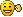I wish I could send you two a cuppa tea and a biscuit in thanks.
YAY PYTHON \o/

In Josh We Trust
-=326.3827=-
Post

### Re: How to program in Python + Paste Code for review/help

#50
I'm reading the following (very advanced, I know), but it doesn't show anything for mePython Memory Management

So I created sizeof.py as follows:

Code: Select all

``````import sys

def show_sizeof(x, level=0):

print "\t" * level, x.__class__, sys.getsizeof(x), x

if hasattr(x, '__iter__'):
if hasattr(x, 'items'):
for xx in x.items():
show_sizeof(xx, level + 1)
else:
for xx in x:
show_sizeof(xx, level + 1)

show_sizeof(None)
show_sizeof(3)
show_sizeof(2**63)
show_sizeof(102947298469128649161972364837164)
show_sizeof(918659326943756134897561304875610348756384756193485761304875613948576297485698417)
``````
And when I run it, I get no response:
p:\>.run-python-script.cmd sizeof.py

p:\>
What I should see:
8 None
12 3
22 9223372036854775808
28 102947298469128649161972364837164
48 918659326943756134897561304875610348756384756193485761304875613948576297485698417

Josh, any snippets on how LT manages memory?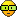I got it working by removing the Carriage returns and line feeds that were copied from the browser.
derp!

The responses I get are:
p:\>.run-python-script.cmd sizeof.py
<type 'NoneType'> 16 None
<type 'int'> 24 3
<type 'long'> 36 9223372036854775808
<type 'long'> 40 102947298469128649161972364837164
<type 'long'> 60 918659326943756134897561304875610348756384756193485761304875613948576297485698417
And in case you're wondering, .run-python-script.cmd contains:

Code: Select all

``````p:\>type .run-python-script.cmd
@python %1 %2 %3 %4 %5 %6 %7 %8 %9
``````
Last edited by FormalMoss on Fri Apr 15, 2016 12:29 pm, edited 1 time in total.
YAY PYTHON \o/

In Josh We Trust
-=326.3827=-
Post

### Re: How to program in Python + Paste Code for review/help

#51
Just to be certain, you did add

Code: Select all

``````show_sizeof(None)
show_sizeof(3)
show_sizeof(2**63)
show_sizeof(102947298469128649161972364837164)
show_sizeof(918659326943756134897561304875610348756384756193485761304875613948576297485698417)``````
to the bottom of sizeof.py right?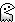Edit: derp, didn't see the scrollbar. Nevermiiiiind
Edit 2: works for meThe LT IRC / Alternate link || The REKT Wiki || PUDDING
Post

### Re: How to program in Python + Paste Code for review/help

#52
This took me ages, but hey, I'm over the moonSpoiler:      SHOW

Code: Select all

``````
# Version.txt contains a simple number, 1
_file = 'Version.txt'

# Open the file and print its contents to the user
file_name = open(_file)
print ""
print "Old Version: %s " % file_name.read()

# Ask the user for a new version number
Custom_Version = raw_input("Current Version Number: ")

# open the file in "write" mode
file_name = open(_file, 'w')
# Write the number entered by the user to the file
file_name.write(Custom_Version)
# NB: Close the file
file_name.close()

print ""
print "Alright, all done."
``````
And the script in action:
Spoiler:      SHOW
PS P:\_ex17.py> ..\.run-python-script.cmd .\ex17-ver3.py

Old Version: 3
Current Version Number: 5

Alright, all done.
PS P:\_ex17.py>
PS P:\_ex17.py>
PS P:\_ex17.py> ..\.run-python-script.cmd .\ex17-ver3.py

Old Version: 5
Current Version Number: 2

Alright, all done.
PS P:\_ex17.py> ..\.run-python-script.cmd .\ex17-ver3.py

Old Version: 2
Current Version Number: 3

Alright, all done.
PS P:\_ex17.py>
Last edited by FormalMoss on Fri Apr 15, 2016 10:29 pm, edited 1 time in total.
YAY PYTHON \o/

In Josh We Trust
-=326.3827=-
Post

### Re: How to program in Python + Paste Code for review/help

#53
3 hours later, and I'm feeling like I know what Josh is talking about when it comes to graphics.. time fliesbut very little headwaySo, in this code, Version.txt contains a number: 1

Python file: IncrementVersion-Auto.py
Spoiler:      SHOW

Code: Select all

``````n = 0
print n

_file = 'Version.txt'

def Auto_ver():
global n
for line in open(_file):
if line.strip():
n = int(line)
print "Inside Loop (n), n = %i" %n
n += 1
print "Inside Loop (n+1), n = %i" % n

Auto_ver()
print ""
print "Outside Loop (n+1), n = %i" %n

print ""
file_name = open(_file)
print "Contents of Version.txt: %s" % file_name.read()
file_name.close()

# file_name = open(_file, 'w')
# file_name.write(n)
# file_name.close()

print ""
print "Alright, all done."
``````
The results work, but it doesn't update Variable.txt:
Spoiler:      SHOW
p:\_ex17.py>type Version.txt
1

p:\_ex17.py>..\.run-python-script.cmd test.py
0
Inside Loop (n), n = 1
Inside Loop (n+1), n = 2

Outside Loop (n+1), n = 2

Contents of Version.txt: 1

Alright, all done.

p:\_ex17.py>type Version.txt
1
I have tried adding extra lines to open the file with 'w'rite options, but the value ends up being empty, and Variable.txt is updated as ' '.

Code: Select all

``````file_name = open(_file, 'w')
file_name.write(int(n))
file_name.close()
``````
And these are the results:
Spoiler:      SHOW
p:\_ex17.py>type Version.txt
1
p:\_ex17.py>..\.run-python-script.cmd test.py
0
Inside Loop (n), n = 1
Inside Loop (n+1), n = 2

Outside Loop (n+1), n = 2

Contents of Version.txt: 1
Traceback (most recent call last):
File "test.py", line 26, in <module>
file_name.write(n)
TypeError: expected a character buffer object

p:\_ex17.py>type Version.txt

p:\_ex17.py>
EDIT: Yay, I fixed this error: ValueError: invalid literal for int() with base 10: '\xff\xfe2'
Turns out if Version.txt contains any special characters, it gives this error.
Notepad doesn't fix it, but a custom shell editor (bbnote.exe, from blackbox window manager), sees these extra characters and I can remove them.

EDIT2: And I fixed it! It works as intended - wow - what a rite of passage that was!

File: Version.txt, contains a value of 24
File: test.py
Spoiler:      SHOW

Code: Select all

``````
n = 0
print ""
print "Initial Value of n: %i" % n

_file = 'Version.txt'

def Increment_Version():
global n
for line in open(_file):
if line.strip():
n = int(line)
print "Inside Loop (Read from _file), n = %i" %n
n += 1
print "Inside Loop (n+1), n = %i" % n

Increment_Version()
print ""
print "Outside Loop (n+1), n = %i" %n

print ""
file_name = open(_file)
print "Contents of Version.txt (Before Write): %s" % file_name.read()

Output = str(n)

file_name = open(_file, 'w')
file_name.write(Output)
file_name.close()

print ""
file_name = open(_file)
print "Contents of Version.txt (After Write): %s" % file_name.read()

print ""
print "Alright, all done."
file_name.close()

# import os
# file_name.seek(0, os.SEEK_CUR)
``````
And the Results:
Spoiler:      SHOW
p:\_ex17.py>..\.run-python-script.cmd test.py

Initial Value of n: 0
Inside Loop (Read from _file), n = 24
Inside Loop (n+1), n = 25

Outside Loop (n+1), n = 25

Contents of Version.txt (Before Write): 24

Contents of Version.txt (After Write): 25

Alright, all done.

p:\_ex17.py>..\.run-python-script.cmd test.py

Initial Value of n: 0
Inside Loop (Read from _file), n = 25
Inside Loop (n+1), n = 26

Outside Loop (n+1), n = 26

Contents of Version.txt (Before Write): 25

Contents of Version.txt (After Write): 26

Alright, all done.

p:\_ex17.py>
YAY PYTHON \o/

In Josh We Trust
-=326.3827=-
Post

### Re: How to program in Python + Paste Code for review/help

#54
How to program in functions, answer to Chapter 21 from
Learn Python The Hard Way - Chapter 21 - Functions can return something
Spoiler:      SHOW

Code: Select all

``````def add (a,b):
# print "ADDING %d + %d" % (a,b)
return a + b

def subtract(a,b):
# print "SUBTRACTING %d - %d " % (a,b)
return a - b

def multiply(a,b):
# print "MULTIPLYING %d * %d " % (a,b)
return a * b

def divide(a,b):
# print "DIVIDING %d / %d " % (a,b)
return a / b

def puzzle(age, height, weight, iq):
total = divide(iq, 2)
total = multiply(weight, total)
total = subtract(height, total)

#####################
#  Start of Program #
print ""
print "Let's do some math with just functions!"

#######################################################
# This is where the action happens, setting variables #
height = subtract(78, 4)
weight = multiply(90, 2)
iq = divide(100, 2)

#######################################################
# Both of these work the same, just one is easier to  #
# read and uses a function \o/                        #
what = puzzle(age, height, weight, iq)
# what = add(age, subtract(height, multiply(weight, divide(iq, 2))))

print ""
print "Age: %d, Height: %d, Weight: %d, IQ: %d" % (age, height, weight, iq)

# A puzzle for the extra credit, type it in anyway.
print ""
print "Here is a puzzle."
print ""
print "That becomes: ", what, "Can you do it by hand?"

#######################################################

``````
YAY PYTHON \o/

In Josh We Trust
-=326.3827=-
Post

### Re: How to program in Python + Paste Code for review/help

#55
About time I updated this.. I've since moved over to Linux for programming in Python, as the Windows box, is well, more an oversized video player (and hooked up to a plasma tv, instead of PC monitor).

I'm quite amazed I forgot how to perform a simple print statement in Python, but here we go, this worked.. although I don't know what repr means just yet.

Code: Select all

``````#!/usr/bin/python

a=1
output = 'a is ' + repr(a)
print (output)
print ""

b=2
output = 'b is ' + repr(b)
print (output)
print ""

c=a+b
output = 'c is ' + repr(c)
print (output)
print ""
#print c

``````
Basically, I found it difficult at first to print a str and int on the same line.. this seems to work until I get better at this.

P.S. What is the use of icode?
YAY PYTHON \o/

In Josh We Trust
-=326.3827=-
Post

### Re: How to program in Python + Paste Code for review/help

#56
What version of Python are you using? It seems that you are mixing 3.x and 2.x syntax for print statements. In Python 3.x you have to use the brackets, so i am going to guess that you are using 2.7.
I have basically no experience with 2.x as it is outdated and no longer useful to learn over 3.x, but in 3 you can use the print statement as follows:
`print(stuffToPrint1,stuffToPrint2, ..., stuffToPrintn)`
to print the n things. With the optional parameter sep you can even set how they should be separated on printing.
`print(stuffToPrint1,stuffToPrint2, ..., stuffToPrintn, sep=";")`
This prints everything with a semicolon between them. Default is a space.

You can use icode to mark as code in a line as I did abovePost

### Re: How to program in Python + Paste Code for review/help

#57
thedamngod wrote:
Sat Jan 27, 2018 12:07 pm
What version of Python are you using?
Thank you TDG, I was wondering why it had changed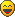I'm using 2.7.12, with GCC 5.4.0 on linux2.

I'm happy to keep with 2.7, work uses this version too, and it looks like I'm going to have to start from Goog-Scratch.. with a penchant for 2.7.What have you programmed in python 3?
YAY PYTHON \o/

In Josh We Trust
-=326.3827=-
Post

### Re: How to program in Python + Paste Code for review/help

#58
So, after a few minutes I've figured out how to create a function called "dbprint", but I don't know how to ask what I'm looking for.

The code is below, but the output shows:
Variable 'x' has a value of 1 <-- this is a
Variable 'x' has a value of 2 <-- this is b
Variable 'x' has a value of 3 <-- this is c
The values are correct, but I don't know how to print out a, b, c where 'x' is shown.
I can print the value of a, b or c, just not the Variable.

Code: Select all

``````#!/usr/bin/python

def dbprint(x):
print "Variable 'x' has a value of %s" % (x)

a=1
dbprint(a)

b=2
dbprint(b)

c=a+b
dbprint(c)
``````
YAY PYTHON \o/

In Josh We Trust
-=326.3827=-
Post

### Re: How to program in Python + Paste Code for review/help

#59
I guess what you are looking for is a debug print function?!
My short research has shown that this might be not an easy problem also regarding safety of the code, but for now this might work:

Code: Select all

``````def dbprint(x):
print 'Variable', x, 'has a value of', repr(eval(x))
``````
Then you should use it like

Code: Select all

``````a = 1
dbprint('a')
``````
Note the single quotes around the a. Remember that this is very likely something you don't want to use in production code and is probably a very bad practice and style.

I havn't worked much in Python in general except for some course work and very small projects.
Post

### Re: How to program in Python + Paste Code for review/help

#60
thedamngod wrote:
Sat Jan 27, 2018 5:18 pm
I guess what you are looking for is a debug print function?!
I have no idea what this means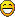Anyways, I found what I was looking for, and this is it!!This is version 2.7 of python.

Code: Select all

``````#!/usr/bin/python

import inspect

x,y = 1,2
z = x+y

def retrieve_name(var):
callers_local_vars = inspect.currentframe().f_back.f_locals.items()
test = [var_name for var_name, var_val in callers_local_vars if var_val is var]
print "%s is %s" % (test, var)

retrieve_name(x)
retrieve_name(y)
retrieve_name(z)
``````
The output is as follows:
``` ['x'] is 1 ['y'] is 2 ['z'] is 3 ```

So, what I wanted was a simple way to send the both the container name and content of that container (i.e. the variable) to a function, once!
So the variable being actioned upon was both the container name AND the variable.
Simples!

Ha ha!
Similar code for Python 3.5!!Code: Select all

``````#!/usr/bin/python3

import inspect

x, y = 1, 2
z = x + y

def retrieve_name(var):
callers_local_vars = inspect.currentframe().f_back.f_locals.items()
test = [var_name for var_name, var_val in callers_local_vars if var_val is var]
print(test, " has a value of ", var)

retrieve_name(x)
retrieve_name(y)
retrieve_name(z)

``````
Output:
``` ['x'] has a value of 1 ['y'] has a value of 2 ['z'] has a value of 3 ```
YAY PYTHON \o/

In Josh We Trust
-=326.3827=-

### Online Now

Users browsing this forum: No registered users and 1 guest